# Modeling an Anti-Lock Braking System

This example shows how to model a simple model for an Anti-Lock Braking System (ABS). It simulates the dynamic behavior of a vehicle under hard braking conditions. The model represents a single wheel, which may be replicated a number of times to create a model for a multi-wheel vehicle.

This model uses the signal logging feature in Simulink®. The model logs signals to the MATLAB® workspace where you can analyze and view them. You can view the code in ModelingAnAntiLockBrakingSystemExample.m to see how this is done.

In this model, the wheel speed is calculated in a separate model named sldemo_wheelspeed_absbrake. This component is then referenced using a 'Model' block. Note that both the top model and the referenced model use a variable step solver, so Simulink will track zero-crossings in the referenced model.

### Analysis and Physics

The wheel rotates with an initial angular speed that corresponds to the vehicle speed before the brakes are applied. We used separate integrators to compute wheel angular speed and vehicle speed. We use two speeds to calculate slip, which is determined by Equation 1. Note that we introduce vehicle speed expressed as an angular velocity (see below).Equation 1From these expressions, we see that slip is zero when wheel speed and vehicle speed are equal, and slip equals one when the wheel is locked. A desirable slip value is 0.2, which means that the number of wheel revolutions equals 0.8 times the number of revolutions under non-braking conditions with the same vehicle velocity. This maximizes the adhesion between the tire and road and minimizes the stopping distance with the available friction.

### Modeling

The friction coefficient between the tire and the road surface, mu, is an empirical function of slip, known as the mu-slip curve. We created mu-slip curves by passing MATLAB variables into the block diagram using a Simulink lookup table. The model multiplies the friction coefficient, mu, by the weight on the wheel, W, to yield the frictional force, Ff, acting on the circumference of the tire. Ff is divided by the vehicle mass to produce the vehicle deceleration, which the model integrates to obtain vehicle velocity.

In this model, we used an ideal anti-lock braking controller, that uses 'bang-bang' control based upon the error between actual slip and desired slip. We set the desired slip to the value of slip at which the mu-slip curve reaches a peak value, this being the optimum value for minimum braking distance (see note below.).

• Note: In an actual vehicle, the slip cannot be measured directly, so this control algorithm is not practical. It is used in this example to illustrate the conceptual construction of such a simulation model. The real engineering value of a simulation like this is to show the potential of the control concept prior to addressing the specific issues of implementation.

### Opening the Model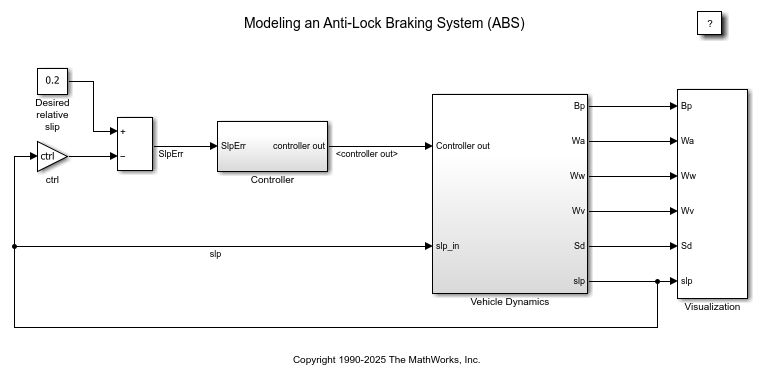Double click on the Wheel Speed subsystem in the model window to open it. Given the wheel slip, the desired wheel slip, and the tire torque, this subsystem calculates the wheel angular speed.To control the rate of change of brake pressure, the model subtracts actual slip from the desired slip and feeds this signal into a bang-bang control (+1 or -1, depending on the sign of the error). This on/off rate passes through a first-order lag that represents the delay associated with the hydraulic lines of the brake system. The model then integrates the filtered rate to yield the actual brake pressure. The resulting signal, multiplied by the piston area and radius with respect to the wheel (Kf), is the brake torque applied to the wheel.

The model multiplies the frictional force on the wheel by the wheel radius (Rr) to give the accelerating torque of the road surface on the wheel. The brake torque is subtracted to give the net torque on the wheel. Dividing the net torque by the wheel rotational inertia, I, yields the wheel acceleration, which is then integrated to provide wheel velocity. In order to keep the wheel speed and vehicle speed positive, limited integrators are used in this model.

### Running the Simulation in ABS Mode

On the Simulation tab, click Run to run the simulation. You can also run the simulation by executing the sim('sldemo_absbrake') command in MATLAB. ABS is turned on during this simulation.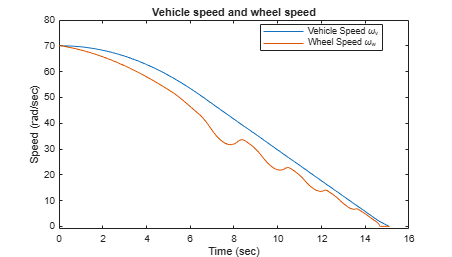• Note: The model logs relevant data to MATLAB workspace in a structure called sldemo_absbrake_output. Logged signals have a blue indicator. In this case yout and slp are logged. Read more about Signal Logging in Simulink Help.

The plots above show the ABS simulation results (for default parameters). The first plot shows the wheel angular velocity and corresponding vehicle angular velocity. This plot shows that the wheel speed stays below vehicle speed without locking up, with vehicle speed going to zero in less than 15 seconds.

### Running the Simulation Without ABS

For more meaningful results, consider the vehicle behavior without ABS. At the MATLAB command line, set the model variable ctrl = 0. This disconnects the slip feedback from the controller, resulting in maximum braking.

ctrl = 0;

Now run the simulation again. This will model braking without ABS.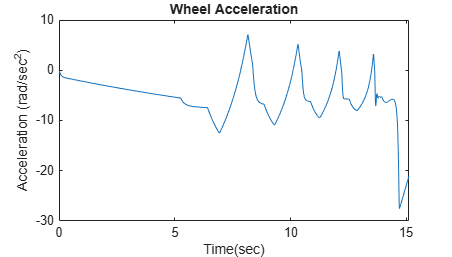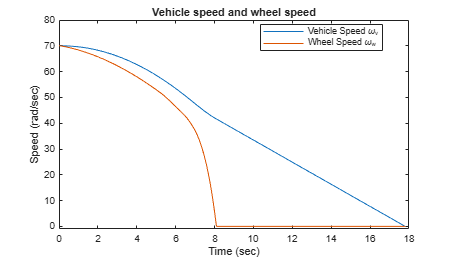### Braking With ABS Versus Braking Without ABS

In the plot showing vehicle speed and wheel speed, observe that the wheel locks up in about seven seconds. The braking, from that point on, is applied in a less-than-optimal part of the slip curve. That is, when slip = 1, as the slip plot shows, the tire is skidding so much on the pavement that the friction force has dropped off.

This is, perhaps, more meaningful in terms of the comparison shown below. The distance traveled by the vehicle is plotted for the two cases. Without ABS, the vehicle skids about an extra 100 feet, taking about three seconds longer to come to a stop.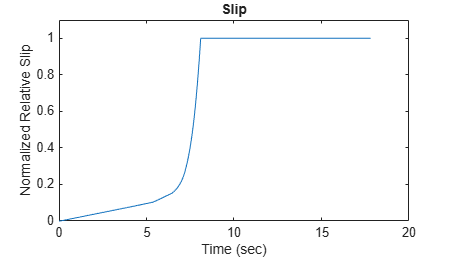### Closing the Model

Close the model. Close the 'Wheel Speed' subsystem. Clear logged data.

### Conclusions

This model shows how you can use Simulink to simulate a braking system under the action of an ABS controller. The controller in this example is idealized, but you can use any proposed control algorithm in its place to evaluate the system's performance. You can also use the Simulink® Coder™ with Simulink as a valuable tool for rapid prototyping of the proposed algorithm. C code is generated and compiled for the controller hardware to test the concept in a vehicle. This significantly reduces the time needed to prove new ideas by enabling actual testing early in the development cycle.

For a hardware-in-the-loop braking system simulation, you can remove the 'bang-bang' controller and run the equations of motion on real-time hardware to emulate the wheel and vehicle dynamics. You can do this by generating real-time C code for this model using the Simulink Coder. You can then test an actual ABS controller by interfacing it to the real-time hardware, which runs the generated code. In this scenario, the real-time model would send the wheel speed to the controller, and the controller would send brake action to the model.